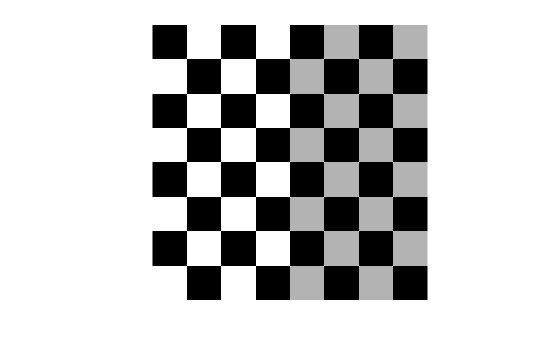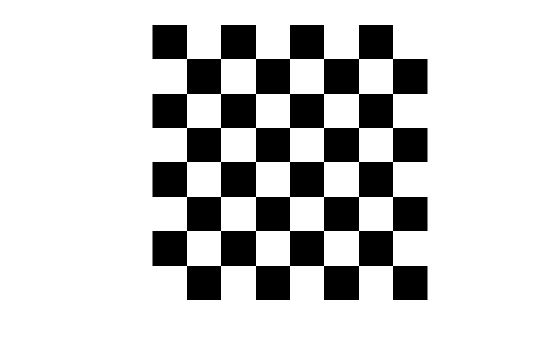# checkerboard

Create checkerboard image

## Syntax

``I = checkerboard``
``I = checkerboard(n)``
``I = checkerboard(n,p,q)``

## Description

example

````I = checkerboard` creates an 8-by-8 square checkerboard image that has four identifiable corners. The checkerboard pattern is made up of tiles. Each tile contains four squares, each with a default of 10 pixels per side. The light squares on the left half of the checkerboard are white. The light squares on the right half of the checkerboard are gray.TILE = [DARK LIGHT; LIGHT DARK]```

example

````I = checkerboard(n)` creates an 8-by-8 square checkerboard image where each square has `n` pixels per side.```

example

````I = checkerboard(n,p,q)` creates a rectangular checkerboard image where `p` specifies the number of rows of tiles and `q` specifies the number of columns of tiles. If you omit `q`, the number of columns defaults to `p` and the checkerboard is square. Each square has `n` pixels per side.```

## Examples

collapse all

Create a checkerboard where the side of every square is 20 pixels in length.

`I = checkerboard(20);`

Display the checkerboard.

`imshow(I)`Create a rectangular checkerboard that is 2 tiles high and 3 tiles wide. The side of every square is 20 pixels in length.

`J = checkerboard(20,2,3);`

Display the checkerboard.

```figure imshow(J)```Create a black and white checkerboard with the default tile size and the default number of rows and columns.

`K = (checkerboard > 0.5);`

Display the checkerboard.

```figure imshow(K)```## Input Arguments

collapse all

Side length in pixels of each square in the checkerboard pattern, specified as a positive integer.

Number of rows of tiles in the checkerboard pattern, specified as a positive integer. Since there are four squares per tile, there are `2*p` rows of squares in the checkerboard.

Number of columns of tiles in the checkerboard pattern, specified as a positive integer. If you omit `q`, the value defaults to `p` and the checkerboard is square. Since there are four squares per tile, there are `2*q` columns of squares in the checkerboard.

## Output Arguments

collapse all

Rectangular image with a checkerboard pattern, returned as a 2-D numeric array. The light squares on the left half of the checkerboard are white. The light squares on the right half of the checkerboard are gray.

Data Types: `double`

## Version History

Introduced before R2006a